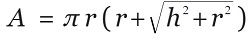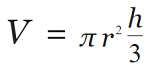# C program to calculate the surface area, volume of Cone

Here, we are going to learn how to calculate the surface area, volume of Cone using C program?
Submitted by Nidhi, on August 10, 2021

Problem Solution:

Read the height and radius of a Cone from the user, and then calculate the surface area and volume of Cone.

Surface area of Cone:

To calculate the surface area of Cone (Right Circular Cone), the formula is:Volume of Cone:

To calculate the volume of Cone (Right Circular Cone), the formula is:Where,

• r : Radius of the Cone
• h : Height of the Cone

Program:

The source code to calculate the surface area, volume of the Cone is given below. The given program is compiled and executed using GCC compile on UBUNTU 18.04 OS successfully.

```// C program to find the surface Area and
// volume of Cone

#include <stdio.h>
#include <math.h>

int main()
{
float height = 0;

float volume = 0;
float area = 0;

printf("Enter the height: ");
scanf("%f", &height);

volume = (1.0 / 3) * (3.14) * radius * radius * height;

printf("Volume of Cone 	    : %f\n", volume);
printf("Surface area of Cone: %f\n", area);

return 0;
}
```

Output:

```RUN 1:
Enter the height: 12.3
Volume of Cone      : 205.984009
Surface area of Cone: 212.691849

RUN 2:
Enter the height: 1.2
Volume of Cone      : 14.519361
Surface area of Cone: 74.791267

RUN 3:
Enter the height: 10
Volume of Cone      : 376.799988
Surface area of Cone: 332.750275
```

Explanation:

Read the value of height and radius from the user. Then we calculated the surface area and volume of Cone using standard formulas.